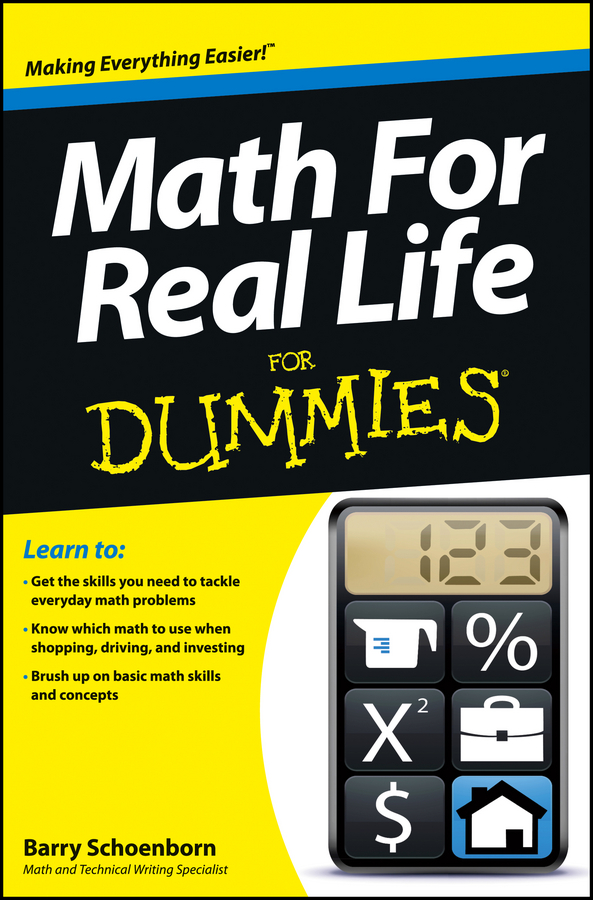##### Math For Real Life For DummiesWhether you're leaving a tip at a restaurant or figuring out just how much those stylish shoes are on sale, you can't get away from percentages. While there are numerous percentage calculators online, it's helpful to be able to do some quick math in your head to calculate percentages without any digital assistance.

## What is percentage?

The word percentage comes from the word percent. If you split the word percent into its root words, you see “per” and “cent.” Cent is an old European word with French, Latin, and Italian origins meaning “hundred." So, percent is translated directly to “per hundred.” If you have 87 percent, you literally have 87 per 100. If it snowed 13 times in the last 100 days, it snowed 13 percent of the time.

## How to find percentage

The numbers that you will be converting into percentages can be given to you in two different formats: decimal and fraction. Decimal format is easier to calculate into a percentage. Converting a decimal to a percentage is as simple as multiplying it by 100. To convert .87 to a percent, simply multiply .87 by 100.

.87 × 100=87, which gives us 87 percent.

Percent is often abbreviated with the % symbol. You can present your answer as 87% or 87 percent — either way is acceptable.

If you are given a fraction, convert it to a percentage by dividing the top number by the bottom number. If you are given 13/100, you would divide 13 by 100.

13 ÷ 100 = .13

Then, follow the steps above for converting a decimal to a percent.

.13 × 100 = 13, thus giving you 13%.

The more difficult task comes when you need to know a percentage when you are given numbers that don’t fit so neatly into 100.

Most of the time, you will be given a percentage of a specific number. For example, you may know that 40 percent of your paycheck will go to taxes and you want to find out how much money that is.

## How to calculate percentage of a specific number

This process is the reverse of what you did earlier. First convert the percentage number to a decimal. Then, you divide your percentage by 100. So, 40 percent would be 40 divided by 100.

40 ÷ 100 = .40

Next, once you have the decimal version of your percentage, simply multiply it by the given number (in this case, the amount of your paycheck). If your paycheck is \$750, you would multiply 750 by .40.

750 × .40 = 300

Let’s try another example. You need to save 25 percent of your paycheck for the next 6 months to pay for an upcoming vacation. If your paycheck is \$1,500, how much should you save?

Start by converting 25 percent to a decimal.

25 ÷ 100 = .25

Now, multiply the decimal by the amount of your paycheck, or 1500.

1500 × .25 = 375

This means you need to save \$375 from each paycheck.